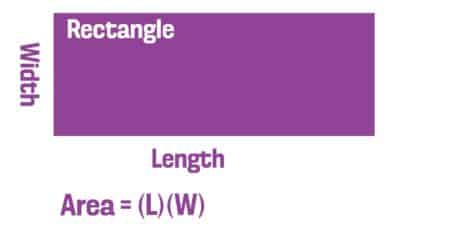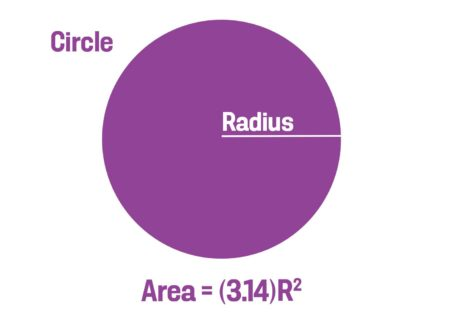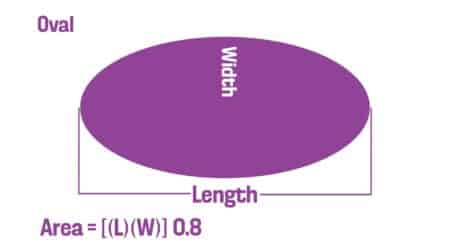Shipping Fast! Delivery in 2-6 business days.

0

# How Many Bulbs Do I Need?

To answer this question, you need to:

• Determine the square footage of the area you are planting. See the formulas provided below.
• Determine the number of bulbs to plant per square foot (the density). We provide a recommended planting density for every item on this website, along with a calculator that will do the math for you.

If you have any questions about calculating square footage or how many bulbs to plant per square foot, don’t hesitate to give us a call.

Please note: Planting is hard work. If you are a landscape professional or you have experience with bulbs, order away. If not, we suggest you order in small bites to learn what it takes to get bulbs planted in the fall. If you like the result in the spring, and you feel you can do more, order more the following fall. This gradual approach will be good for your back, your budget, and your learning curve.

### Rectangle and Square

The area of a rectangle and a square is found by multiplying the length (L) by the width (W).### Circle

The area of a circle is the radius squared (i.e., the radius multiplied by itself) multiplied by pi (~3.14). The radius is equal to one-half the diameter of the circle.### Triangle

The area of a triangle is one-half the base (B) multiplied by the height (H).### Oval

The area of an oval is the length (L) multiplied by the width (W), multiplied by 0.8.### Irregular Shapes

To find the area of a large irregular shape, divide it into a series of smaller units equally spaced along a measured line. This method will calculate the area to within 5 percent.

1. Determine the axis line. This is the longest axis of the shape, shown below as A to B.
2. Mark several perpendicular lines along the axis line to divide it into several segments of equal depth. To ensure accuracy, use as many lines as possible. The more irregular the shape, the more lines you will need.
3. Measure the width of the bed at each perpendicular line.
4. Calculate the area by adding the lengths of all the perpendicular lines and multiplying by the distance between the lines.### Example

The distance between points A and B is 60 feet.

Five perpendicular lines break the A-B line into six segments of equal depth: 10 feet.

The perpendicular lines have the following measurements:

C = 19 feet

D = 22 feet

E = 23 feet

F = 19 feet

G = 13 feet

The approximate area is: (19 + 22 + 23 + 19 + 13) x 10 = 960 sq. ft.

## Recommended Articles

#### Moving and Dividing Daffodils

The ideal time to move or divide daffodils is when the foliage has collapsed and has turned mostly but not completely brown. For most daffodils, this time comes in late spring or early summer.

#### How to Protect Tulip Bulbs from Squirrels

If there are squirrels in your area, it’s best to take precautions to protect your tulip and crocus bulbs after planting.